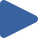# Merge Sort

## Animation, code, analysis, and discussion of merge sort on 4 initial conditions.

How to use: Press "Play all", or choose thebutton.

 Play All Play animationRandomPlay animationNearly SortedPlay animationReversedPlay animationFew Unique### ALGORITHM

``````# split in half
m = n / 2

# recursive sorts
sort a[1..m]
sort a[m+1..n]

# merge sorted sub-arrays using temp array
b = copy of a[1..m]
i = 1, j = m+1, k = 1
while i <= m and j <= n,
a[k++] = (a[j] < b[i]) ? a[j++] : b[i++]
→ invariant: a[1..k] in final position
while i <= m,
a[k++] = b[i++]
→ invariant: a[1..k] in final position
``````

### DISCUSSION

Merge sort is very predictable. It makes between 0.5lg(n) and lg(n) comparisons per element, and between lg(n) and 1.5lg(n) swaps per element. The minima are achieved for already sorted data; the maxima are achieved, on average, for random data. If using Θ(n) extra space is of no concern, then merge sort is an excellent choice: It is simple to implement, and it is the only stable O(n·lg(n)) sorting algorithm. Note that when sorting linked lists, merge sort requires only Θ(lg(n)) extra space (for recursion).

Merge sort is the algorithm of choice for a variety of situations: when stability is required, when sorting linked lists, and when random access is much more expensive than sequential access (for example, external sorting on tape).

There do exist linear time in-place merge algorithms for the last step of the algorithm, but they are both expensive and complex. The complexity is justified for applications such as external sorting when Θ(n) extra space is not available.

### KEY

• Black values are sorted.
• Gray values are unsorted.
• A red triangle marks the algorithm position.
• Dark gray values denote the current interval.

### PROPERTIES

• Stable
• Θ(n) extra space for arrays (as shown)
• Θ(lg(n)) extra space for linked lists
• Θ(n·lg(n)) time